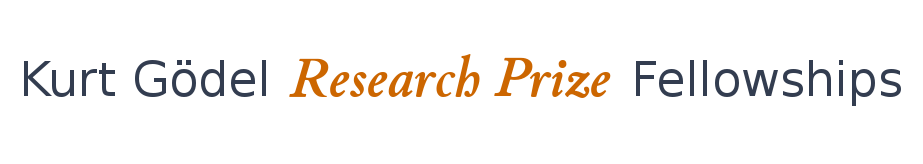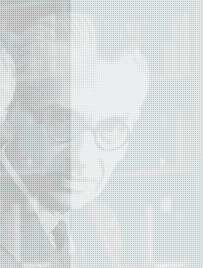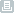HomeFinalists and WinnersPrevious FellowshipsTerms and ConditionsPrint this page

# Model Questions for Logical Foundations of Mathematics

These questions were prepared by Matthias Baaz and Harvey Friedman in accordance with Hyung Choi's questions' catalogue.

## WHAT IS THE PHILOSOPHICAL BASIS FOR LOGIC?

How can we find a new and more convincing way to justify the axioms, or substantial fragments of the axioms, of set theory? Are there good arguments for their internal consistency that don't address their truth? How can this be extended to large cardinal axioms? How can this be extended to other kinds of extensions of the usual axioms for set theory?

How can we give clearer formulations of the main "isms" such as Platonism, realism, formalism, constructivism, empiricism, nominalism, conventionalism, etc., and formulate theorems that attack or defend these positions? (This means understanding logic in Gödel's way: the philosophical analysis is the most important instrument to deal with mathematical reality, however, this instrument is effective for mathematics and philosophy only if philosophical positions are connected to mathematical theorems)

## ARE THERE DIFFERENT LOGICAL SYSTEMS THAT ARE MUTUALLY INCOMPATIBLE?

How to deal with the conflict of deductive logic fundamental for mathematics and sciences, and abductive logic e.g. in legal decisions? (In legal systems decisions have to be made with apriori limited resources. The consistency of such decisions is ensured in an empirical way. From a mathematical viewpoint all such systems are inconsistent, but there is no doubt that they are effective)

Do different sciences relate to different possibly incompatible logics? Is a recognizable failure of mathematization in many fields connected to the unsuitability of classical logic? (The prime example is quantum logic and von Neumann's Theorem of the impossibility to introduce hidden variables.)

## CAN LOGIC BE CONSIDERED EMPIRICAL? CAN LOGICAL PRINCIPLES BE DERIVED FROM PHYSICAL PRINCIPLES?

How can we give a convincing, or more convincing, proof of Church's Thesis? What are the analogues of Church's Thesis for complexity classes such as P, NP, PSPACE, etc.? How can we give a convincing, or more convincing, proof of these forms of Church's Thesis?

Is classical logic a limit case of logics related to the physical world similar to the limit status of the Euclidian space?

## CAN CREATIVITY (OR CREATIVE PROCESSES) BE AUTOMATIZED?

How can we develop a proof theory of analogical reasoning? How can we formalize the abstraction of general statements from "good" examples? (Babylonian mathematicians communicated general facts by examples. Even in contemporary mathematics there is a strong feeling, that good examples comprise the essence of mathematical creativity.)

How can we classify the different levels of obviousness and triviality in mathematics? To what extent can we program computers to recognize these levels using practical resources?

## CAN LOGICAL SYSTEMS BE CONSTRUCTED DIFFERENTLY FOR DIFFERENT TYPES OF WORLDS OR DIFFERENT WORLDS OF EXPERIENCE?

Should logic depend on the assumption of a common world of experience? Intuitionistic logic corresponds to the cancellation of this assumption. How can we give a convincing, or more convincing completeness proof for intuitionistic logic that better explains the naturalness of the usual axioms and rules?

Choice sequences are at the core of intuitionism. They are formulated as a concept of real numbers, however they relate to all objects if one considers objects as given by their step-by-step recognized properties. Is intuitionism a better foundation than classical mathematics for sciences, where general assumptions are problematic?

## ARE THERE FUNDAMENTAL LIMITS OF LOGICS AND MATHEMATICS IN DESCRIBING THE REAL WORLD?

In what sense and to what extent does reading a proof or interaction with mathematicians provide absolute certainty about the result? In what sense and to what extent does interaction with proof assistants provide absolute certainty about the result?

Is there a general way to define what classification means in core mathematics? E.g., the classification of finite Abelian groups, 2- manifolds, finite simple groups, etc. Can we show that in interesting cases, in core mathematics, classifications do not exist?

## WHAT ARE THE MOST FUNDAMENTAL ASSUMPTIONS IN MATHEMATICS AND LOGIC?

How can we deal with Brower's doubt in his early writings: "Are we sure, that the repeated applications of formal rules do not change their meaning?" Is syntax without elementary induction possible and reasonable?

How can we make logic less circular? How can we identify a core circularity that cannot be removed?

## WHAT IS A PROOF? WHAT IS THE RELATIONSHIP BETWEEN THE IDEAS OF PROOFS AND TRUTH?

The most important but least understood concept of mathematics is the concept of proof. When proofs are equal (i.e. which transformations are invariant on proofs)? Under which circumstances can proofs be represented by examples (as Babylonians did)? Are proofs objects independent of the human mind as Erdös suggested?

What more do we know given the proof of a statement than simply knowing that the statement is true? (This question by Georg Kreisel possibly describes the essence of proof theory.)

## IS MATHEMATICS CONSTRUCTED OR DISCOVERED?

If we assume that mathematics is constructed, a coherence of proof and construction should exist. Do the Incompleteness Theorems lead to nonconstructable objects and thereby refute this assumption? Are there suitable transfinite constructions not sensitive to the theorems mentioned?

Is it really possible to develop a method for discovery of new axioms in set theory using Husserl's Phenomenology as claimed by Kurt Gödel?

## HOW DO FINITENESS AND INFINITENESS INTERPLAY IN MATHEMATICS?

How can we develop recursion theory for the finite subsets of N? For the subsets of {0,1,...,21000}? For the subsets of {0,1,...,1000}?

The o-minimality concept asserts that every definable subset of the (linearly ordered) domain is a finite union of intervals with endpoints in the domain (with +-infinity). There is a very limited supply of o-minimal structures in mathematics. Can we productively create a theory based on the weakened condition that every n quantifier definable subset is a finite union of intervals with endpoints in the domain (with +- infinity)? (Tameness is a principal seminal theme in model theory. Generally speaking, a structure is tame if and only if its definable relations are "well behaved". The o-minimality concept is a primary example of a tameness notion that is very well studied. The definable subsets are structured by finite means, the underlying (uncountable) infinite is controlled.)

## WHAT IS THE RELATIONSHIP BETWEEN RANDOMNESS AND INFINITE?

Can we integrate the probabilistic and recursion theoretic features of random sequences? Is randomness a tool for better computing? Is randomness useful for establishing formal concepts of learning and creativity?

Forcing was originally introduced as a technical tool by Paul Cohen in order to obtain dramatic results about set theory. Today, it is used in the formulation of interesting further results. How can we establish that forcing is of fundamental intrinsic significance?

## SHOULD CANTOR'S IDEA OF THE "COMPLETED INFINITY" BE CONSIDERED SELF EVIDENT?

Large cardinals are considered to be "complete" for the projective hierarchy of sets of reals. How can we state and prove this rigorously? (Cantor's idea of the completed infinity cannot be considered self evident as long the mathematical consequences are not obvious. The projective hierarchy of reals is a prime example where there is the impression of large cardinals being complete, although it is difficult to substantiate this impression formally.)

The formal assumption of a completion within the theory leads to set theories not compatible with ZF (Zermelo-Fraenkel set theory). The most prominent set theory of this kind is Quine's NF(New Foundation). Can we construct adequate semantics for NF and thereby prove its full consistency?